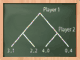Today, part of my teaching concerned basic properties of the total variation on discrete spaces. Let ${E}$ be a possibly infinite countable set equipped with its discrete topology and ${\sigma}$-field. Let ${\mathcal{P}}$ be the set of probability measures on ${E}$. We equip ${\mathcal{P}}$ with the total variation distance defined for every ${\mu,\nu\in\mathcal{P}}$ by

$d_{TV}(\mu,\nu)=\sup_{A\subset{E}}|\mu(A)-\nu(A)|.$

It takes its values in ${[0,1]}$ and ${1}$ is achieved when ${\mu}$ and ${\nu}$ have disjoint supports.

Theorem 1 (Alternative expressions) For every ${\mu,\nu\in\mathcal{P}}$ we have

$d_{TV}(\mu,\nu) =\frac{1}{2}\sup_{f:E\rightarrow[-1,1]}\left|\int\!f\,d\mu-\int\!f\,d\nu\right| =\frac{1}{2}\sum_{x\in E}|\mu(x)-\nu(x)|$

Moreover, the supremum in the original definition of ${d_{TV}}$ is achieved for the set

$A_*=\{x\in E:\mu(x)\geq\nu(x)\}$

while the supremum in the functional expression of ${d_{TV}}$ is achieved for

$f=\mathbf{1}_{A_*}-\mathbf{1}_{A_*^c}.$

Proof: The second equality follows from the inequality

$\left|\int\!f\,d\mu-\int\!f\,d\nu\right| \leq \sum_{x\in E}|f(x)||\mu(x)-\nu(x)| \leq \sup_{x\in E}|f(x)|\sum_{x\in E}|\mu(x)-\nu(x)|$

which is saturated for ${f=\mathbf{1}_{A_*}-\mathbf{1}_{A_*^c}}$. For the first equality, we write

$|\mu(A)-\nu(A)| =\frac{1}{2}\left|\int\!f_A\,d\mu-\int\,f_A\,d\nu\right|$

where ${f=\mathbf{1}_A-\mathbf{1}_{A^c}}$, which gives

$|\mu(A)-\nu(A)| \leq \sup_{f:E\rightarrow[-1,1]}\left|\int\!f\,d\mu-\int\!f\,d\nu\right| = \frac{1}{2}\sum_{x\in E}|\mu(x)-\nu(x)|$

which is saturated for ${A=A_*}$ since

$2|\mu(A_*)-\nu(A_*)|=\sum_{x\in A_*}|\mu(x)-\nu(x)|+\sum_{x\in A_*^c}|\mu(x)-\nu(x)|.$

$\Box$

Theorem 2 (Equivalent criteria for convergence in law) Let ${(X_n)_{n\in\mathbb{N}}}$ be a sequence of random variables on ${E}$ and let ${\mu_n}$ be the law of ${X_n}$. For any ${\mu}$ in ${\mathcal{P}}$, the following properties are equivalent:

1. ${(X_n)_{n\in\mathbb{N}}}$ converges in law to ${\mu}$
2. ${\lim_{n\rightarrow\infty}\int\!f\,d\mu_n=\int\!f\,d\mu}$ for all bounded ${f:E\rightarrow\mathbb{R}}$
3. ${\lim_{n\rightarrow\infty}\mu_n(x)=\mu(x)}$ for all ${x\in E}$
4. ${\lim_{n\rightarrow\infty}d_{TV}(\mu_n,\mu)=0}$

Proof: To deduce 1. from 4. it suffices to use the functional variational expression of ${d_{TV}}$. The properties 1. and 2. are equivalent since every ${f:E\rightarrow\mathbb{R}}$ is continuous for the discrete topology. To deduce 3. from 2. one can take ${f=\mathbf{1}_{\{x\}}}$. To deduce 4. from 3. we start by observing that for an arbitrary ${A\subset E}$,

$\sum_{x\in E}|\mu_n(x)-\mu(x)| =\sum_{x\in A}|\mu_n(x)-\mu(x)| +\sum_{x\in A^c}|\mu_n(x)-\mu(x)|.$

Thanks to 3. for every ${\varepsilon’>0}$ there exists an integer ${N=N(A,\varepsilon’)}$ such that the first term of the right hand side is bounded above by ${\mathrm{card}(A)\varepsilon’}$ for all ${n\geq N}$. For the second term of the right hand side, we write

$\sum_{x\in A^c}|\mu_n(x)-\mu(x)| \leq \sum_{x\in A^c}\mu_n(x)+\sum_{x\in A^c}\mu(x).$

Since we have

$\sum_{x\in A^c}\mu_n(x) =\sum_{x\in A}\mu(x)-\sum_{x\in A}\mu_n(x)+\sum_{x\in A^c}\mu(x)$

we obtain

$\sum_{x\in A^c}|\mu_n(x)-\mu(x)| \leq \sum_{x\in A}|\mu_n(x)-\mu(x)|+2\sum_{x\in A^c}\mu(x).$

Since ${\nu\in\mathcal{P}}$, for any ${\varepsilon”>0}$, we can select ${A}$ finite such that ${\mu(A^c)\leq\varepsilon”}$.$\Box$

Theorem 3 (Yet another expression and the extremal case) For every ${\mu,\nu\in\mathcal{P}}$ we have

$d_{TV}(\mu,\nu)=1-\sum_{x\in E}(\mu(x)\wedge\nu(x)).$

In particular, ${d_{TV}(\mu,\nu)=1}$ if and only if ${\mu}$ and ${\nu}$ have disjoint supports.

Proof: It suffices to write

$\sum_{x\in E}(\mu(x)\wedge\nu(x)) =\frac{1}{2}\sum_{x\in E}(\mu(x)+\nu(x)-|\mu(x)-\nu(x)|)=1-d_{TV}(\mu,\nu).$

$\Box$

Theorem 4 (Coupling) For every ${\mu,\nu\in\mathcal{P}}$ we have

$d_{TV}(\mu,\nu) =\inf_{(X,Y)}\mathbb{P}(X\neq Y)$

where the infimum runs over all the couples of random variables on ${E\times E}$ with marginal laws ${\mu}$ and ${\nu}$. Moreover, there exists a couple of this type for which the equality is achieved.

Proof: Let ${(X,Y)}$ be a couple of random variables on ${E\times E}$ with marginal laws ${\mu}$ nd ${\nu}$. Since ${\mathbb{P}(X=x,Y=x)\leq \mu(x)\wedge\nu(x)}$ for every ${x\in E}$ we have

$1-d_{TV}(\mu,\nu)=\sum_{x\in E}(\mu(x)\wedge\nu(x)) \geq \sum_{x\in E}\mathbb{P}(X=x,Y=x) =\mathbb{P}(X=Y).$

Therefore, it suffices now to construct a couple ${(X,Y)}$ for which the equality is achieved. Define

$p=1-d_{TV}(\mu,\nu)\in[0,1].$

Case where ${p=0}$. Then ${d_{TV}(\mu,\nu)=1}$ and the supports of ${\mu}$ et ${\nu}$ are disjoints. This gives ${\sum_{x\in E}\mu(x)\nu(x)=0}$. We consider in this case ${(X,Y)}$ where ${X\sim\mu}$ and ${Y\sim\nu}$ are independent:

$\mathbb{P}(X=Y)=\sum_{x\in E}\mu(x)\nu(x)=0.$

Case where ${p=1}$. Then ${d_{TV}(\mu,\nu)=0}$ and thus ${\mu=\nu}$. We can take ${(X,X)}$ where ${X\sim\mu}$.

Case where ${0<p<1}$. Let ${(U,V,W)}$ be a triple of random variables with laws

$p^{-1}(\mu\wedge\nu),\quad (1-p)^{-1}(\mu-(\mu\wedge\nu)),\quad (1-p)^{-1}(\nu-(\mu\wedge\nu))$

(recall that ${p=\sum_{x\in E}(\mu(x)\wedge\nu(x))}$). Let ${B}$ be a Bernoulli random variable, independent of ${(U,V,W)}$, such that ${\mathbb{P}(B=1)=1-\mathbb{P}(B=0)=p}$. If we define ${(X,Y)=(U,U)}$ if ${B=1}$ while ${(X,Y)=(V,W)}$ if ${B=0}$, then ${X\sim\mu}$ and ${Y\sim\nu}$, and since the laws of ${V}$ and ${W}$ have disjoint supports, we have ${\mathbb{P}(V=W)=0}$, and thus

$\mathbb{P}(X=Y)=\mathbb{P}(B=1)=p.$

$\Box$

Since ${\mathbb{P}(X\neq Y)=\mathbb{E}(d(X,Y))}$ for the atomic distance ${d(x,y)=1_{x\neq y}}$ we have

$d_{TV}(\mu,\nu)=\min_{\pi\in\Pi(\mu,\nu)}\int_{E\times E}\!d(x,y)\,d\pi(x,y)$

where ${\Pi(\mu,\nu)}$ is the convex set of probability measures on ${E\times E}$ with marginal laws ${\mu}$ and ${\nu}$. From this point of view, ${d_{TV}}$ is the Wasserstein ${W_1}$ distance on ${\mathcal{P}}$ associated to the atomic distance on ${E}$.

## One Comment

1. Luc 2015-11-22

Bonjour,

For general polish space $E$, can we construct explicitly an optimal coupling as you did in the discrete setting ? Any reference/idea would be enough.